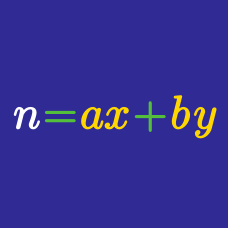Number Theory

Postage Stamp Problem / Chicken McNugget Theorem

A family restaurant sells nuggets in packages of two sizes, 7 nuggets and 12 nuggets. Is there a maximum number of nuggets that $\color{#D61F06}{\text{cannot}}$ be expressed as a nonnegative combination of these package sizes?

Yes or No?

Are there any non-negative integer solutions to the equation

$13m + 15n = 170?$

Yes or No?

If $a>4$ is an integer that is $\color{#D61F06}{\text{not}}$ a multiple of 13, can we express $11a-39$ as a non-negative integer linear combination of $a$ and 13?

In a fast food restaurant, you can order buckets of doughnuts only in quantities of 7 and 19 a bucket. What is the $\color{#D61F06}{\text{second}}$ largest number of doughnuts you $\color{#D61F06}{\text{cannot}}$ buy?

There are only two kinds of postage stamps: 12 cents and 25 cents. For some values, you can get them as linear combinations of two kinds. For others, you cannot. What is the largest possible value you $\color{#D61F06}{\text{cannot}}$ attain no matter which combination you choose? Give your answer in cents.

×